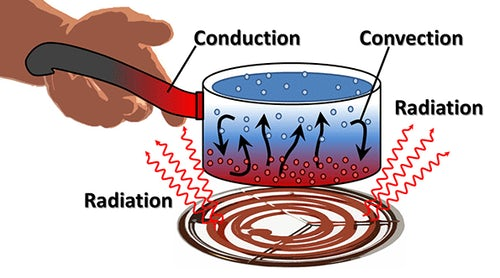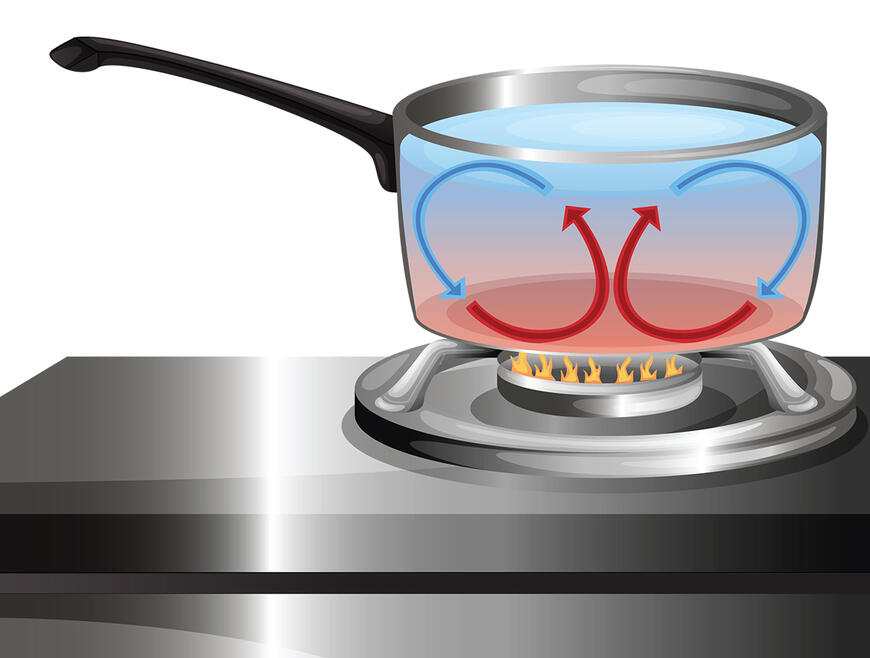11 December 2023
Anasayfa » Types of Heat Transfer

# Types of Heat TransferTypes of Heat Transfer: – There are three basic modes of heat transfer; Conduction, Convection and Radiation.

## 1. Conduction

It is a form of transfer that requires a material medium for heat transfer. This material medium can be solid, liquid or gas.

In solids, if the temperature of a particular part of the material is increased by heat transfer; this means that the internal energy of that part of the material increases. Molecules in that part of the material vibrate back and forth, interacting with neighboring molecules in this process, colliding and transferring energy. Solids also contain free electrons, which are responsible for the conduction of heat as they move from place to place, scatter, and collide with other free electrons.

In liquids and gases, the distance between molecules is greater than in solids. For conduction to occur, the activity of molecules in liquids and gases is such that they do not move too far from their initial position. Due to the large distances between molecules, the extent of conduction is relatively small compared to solids. The rate of heat conduction through a medium depends on the geometry of the object, the temperature difference within the object, and the type of material medium itself.

Fourier’s Law of Heat Conduction

Let’s take as an example A flat plate shown in the figure above, whose two dimensions are quite large relative to its thickness l. Let A be the surface area of the plate, T1 and T2 plate surface temperatures (T1 > T2). The temperature transition will be from the T1 (hot surface) to the T2 (cold) surface. The temperature does not change with time.shown in form. As seen in the expression above, the rate of heat transfer from a solid body (T1-T2) is directly proportional to the temperature difference and surface A, and inversely proportional to the plate thickness L. If the proportionality coefficient k is placed in the above expression,form is obtained. Here k is a property of the material and is defined as the thermal conductivity coefficient. According to the 2nd law of thermodynamics, heat conduction will be in the direction of decreasing temperature. Therefore, the temperature difference and the heat flux have opposite signs. In order to obtain heat transfer in the positive direction, ( – ) sign is placed in front of the above expression. The given expression is actually a definition of the heat transfer coefficient as shown below,can be written as The size of the heat transfer coefficient k is W/mK or W/mC in the SI unit system.

The heat transfer coefficient is the rate of heat transfer transmitted for a unit thickness increase in temperature and varies from material to material. Since its change with pressure is less than its change with temperature, it is neglected. For this reason, the heat transfer coefficient can be considered as a function of temperature. In many cases, the thermal conductivity coefficient changes linearly with temperature as seen in the expression given below.where k0 = k(T0) and T0 is the reference temperature. β is a constant and is called the temperature coefficient of heat conduction. Its unit is 1/K. Heat transfer If the above expression is substituted for k in the Fourier heat conduction law,relation is obtained.

2. Convection

The term convection, in its most general sense, refers to the movement of a fluid. This movement has two components: drift and spread.Newton’s Law of Viscous Voltages

In one dimension for viscous stresses in Newtonian fluids,relation can be written. Here, µ is the dynamic viscosity related to the property of the fluid, y is the distance from the wall, dA is the differential area, dF is the differential shear force, and τ is the shear stress.

Viscosity: It is a measure of the fluid’s resistance to flow. The viscosity of liquids decreases with temperature, while the viscosity of gases increases with temperature. The mass flow rate per unit length is called dynamic viscosity and is denoted by µ. Its unit is Ns/m2 or kg/ms. kinematic viscosity,

given in the form. Its unit is m2/s.

Newton’s Law of Cooling

A fluid at temperature T∞ flows over a solid surface as shown in the figure below. The velocity of the fluid particles in contact with the surface is zero. Other fluid particles slide over stationary particles. Due to the viscous forces, the entrainment of particles in the wall is prevented. The effect of viscous forces decreases from the surface towards the flow center and finally, after the boundary layer thickness, the velocity of the particles reaches the fluid velocity. If there is a temperature difference between the solid surface temperature and the fluid temperature, then there will be heat transfer from the surface to the solid fluid particles by conduction. In this case, the fluid particles on the surface Tw equals the surface temperature and reaches the free flow temperature T∞ asymptotically within the boundary layer thickness and is written as follows.

Here, kf is the heat transfer coefficient of the fluid, Tf is the fluid temperature, n is the direction perpendicular to the surface, and w is the derivative along the surface.

Newton is the heat flux from unit area to unit time between a moving fluid in contact with a solid surface,

given in conjunction. Here Tw is the surface temperature, Tf is the fluid temperature outside the boundary layer, and h is the convection coefficient. This expression is called Newton’s law of cooling.

The calculation of the heat transfer coefficient is quite complicated. It can be found with the help of empirical relations obtained experimentally. The heat transfer coefficient depends on the properties of the fluid such as viscosity, density, heat transmission coefficient, shape of the fluid, flow geometry and speed of the fluid. Its size is W/m2 K in the SI unit system.

The heat radiation coefficient takes different values locally on the surface. In engineering applications, the average heat transfer coefficient across the surface is used. Average heat convection coefficient across the surface,given by the relation.

In heat transfer processes, if the movement of the fluid is carried out with the help of a pump or fan, this is called forced convection. If the fluid movement occurs with the effect of a mass force due to the density difference, this type of heat convection phenomenon is called natural convection. It is written along the surface given by the above equation as follows.

The value of R=1 /hA given in the above expression is called the heat transfer resistance.

Heat transfer by radiation occurs by the emission of electromagnetic waves. These waves carry the energy away from the object from which it is emitted. Radiation occurs through a vacuum or any transparent medium (solid or liquid). Thermal radiation is the direct result of random movements of atoms and molecules in matter. Movements of charged protons and electrons cause electromagnetic waves to propagate.All materials emit thermal energy according to their temperature. The hotter the material, the more thermal energy it emits. The sun is an example of heat radiation that radiates heat throughout the solar system. At normal room temperature, objects propagate as infrared waves. The temperatures of objects also have an effect on the emitted wavelength and frequency. As the temperature increases, the wavelengths of the emitted radiation decrease so that shorter wavelengths with higher frequencies are emitted.

The reflected part (reflectivity) of the radiation coming to the surface is given by the reflection coefficient p , the absorbed part (obsorbivity) absorption coefficient a and the transmitted part (transmissivity) transmission coefficient τ. If the incident radiation is 1 unit

p+a+ τ=1 can be written. For opaque objects, τ = 0. For a black body a = 1 is taken. The surfaces closest to the black body are black carbon and black platinum.

Stefan-Boltzmann Law

The power to emit radiation per unit time per unit area of a surface is proportional to the fourth power of the absolute temperature of the surface. This expression

Eb = σT4

It is given in the form. The relation given by Stefan in 1879 and theoretically obtained by Boltzmann in 1884 is called the Stefan-Boltzmann law. where σ is the Stefan-Boltzmann constant T is the absolute temperature and Eb is the emissivity for the black body. In the SI unit system, it is σ=5,67.10-8 W /M2 K4.